Algebra and Trigonometry

# Review Exercises

Algebra and TrigonometryReview Exercises

### Review Exercises

##### Functions and Function Notation

For the following exercises, determine whether the relation is a function.

1.

${ (a,b),(c,d),(e,d) } { (a,b),(c,d),(e,d) }$

2.

${ (5,2),(6,1),(6,2),(4,8) } { (5,2),(6,1),(6,2),(4,8) }$

3.

$y 2 +4=x, y 2 +4=x,$ for $x x$ the independent variable and $y y$ the dependent variable

4.

Is the graph in Figure 1 a function?

Figure 1

For the following exercises, evaluate $f(−3);f(2);f(−a);−f(a);f(a+h). f(−3);f(2);f(−a);−f(a);f(a+h).$

5.

$f(x)=−2 x 2 +3x f(x)=−2 x 2 +3x$

6.

$f(x)=2| 3x−1 | f(x)=2| 3x−1 |$

For the following exercises, determine whether the functions are one-to-one.

7.

$f(x)=−3x+5 f(x)=−3x+5$

8.

$f(x)=| x−3 | f(x)=| x−3 |$

For the following exercises, use the vertical line test to determine if the relation whose graph is provided is a function.

9.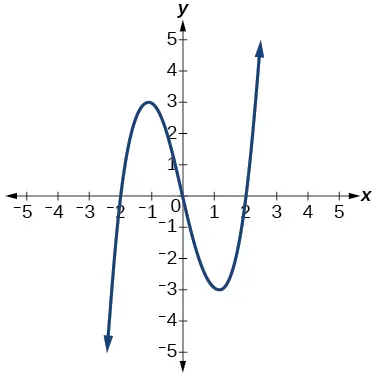10.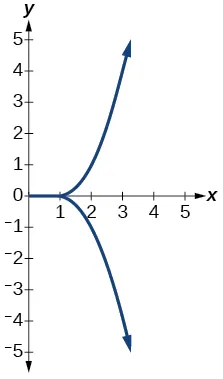11.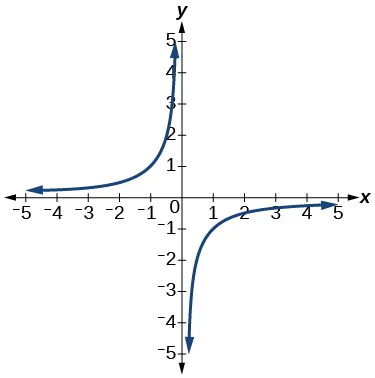For the following exercises, graph the functions.

12.

$f(x)=| x+1 | f(x)=| x+1 |$

13.

$f(x)= x 2 −2 f(x)= x 2 −2$

For the following exercises, use Figure 2 to approximate the values.

Figure 2
14.

$f(2) f(2)$

15.

$f(−2) f(−2)$

16.

If $f(x)=−2, f(x)=−2,$ then solve for $x. x.$

17.

If $f(x)=1, f(x)=1,$ then solve for $x. x.$

For the following exercises, use the function $h(t)=−16 t 2 +80t h(t)=−16 t 2 +80t$ to find the values in simplest form.

18.

$h(2)−h(1) 2−1 h(2)−h(1) 2−1$

19.

$h(a)−h(1) a−1 h(a)−h(1) a−1$

##### Domain and Range

For the following exercises, find the domain of each function, expressing answers using interval notation.

20.

$f(x)= 2 3x+2 f(x)= 2 3x+2$

21.

$f(x)= x−3 x 2 −4x−12 f(x)= x−3 x 2 −4x−12$

22.

$f(x)= x−6 x−4 f(x)= x−6 x−4$

23.

Graph this piecewise function:

##### Rates of Change and Behavior of Graphs

For the following exercises, find the average rate of change of the functions from

24.

$f(x)=4x−3 f(x)=4x−3$

25.

$f(x)=10 x 2 +x f(x)=10 x 2 +x$

26.

$f(x)=− 2 x 2 f(x)=− 2 x 2$

For the following exercises, use the graphs to determine the intervals on which the functions are increasing, decreasing, or constant.

27.28.29.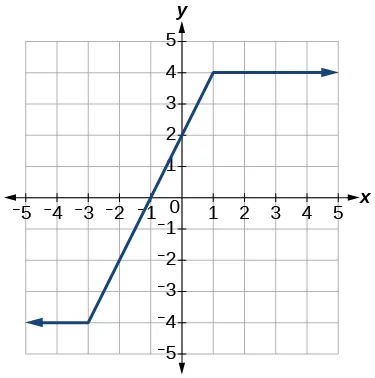30.

Find the local minimum of the function graphed in Exercise 3.27.

31.

Find the local extrema for the function graphed in Exercise 3.28.

32.

For the graph in Figure 3, the domain of the function is $[ −3,3 ]. [ −3,3 ].$ The range is $[ −10,10 ]. [ −10,10 ].$ Find the absolute minimum of the function on this interval.

33.

Find the absolute maximum of the function graphed in Figure 3.

Figure 3
##### Composition of Functions

For the following exercises, find $(f∘g)(x) (f∘g)(x)$ and $(g∘f)(x) (g∘f)(x)$ for each pair of functions.

34.

$f(x)=4−x,g(x)=−4x f(x)=4−x,g(x)=−4x$

35.

$f(x)=3x+2,g(x)=5−6x f(x)=3x+2,g(x)=5−6x$

36.

$f(x)= x 2 +2x,g(x)=5x+1 f(x)= x 2 +2x,g(x)=5x+1$

37.

$f(x)= x+2 ,g(x)= 1 x f(x)= x+2 ,g(x)= 1 x$

38.

$f(x)= x+3 2 ,g(x)= 1−x f(x)= x+3 2 ,g(x)= 1−x$

For the following exercises, find $( f∘g ) ( f∘g )$ and the domain for $( f∘g )(x) ( f∘g )(x)$ for each pair of functions.

39.

$f(x)= x+1 x+4 ,g(x)= 1 x f(x)= x+1 x+4 ,g(x)= 1 x$

40.

$f(x)= 1 x+3 ,g(x)= 1 x−9 f(x)= 1 x+3 ,g(x)= 1 x−9$

41.

$f(x)= 1 x ,g(x)= x f(x)= 1 x ,g(x)= x$

42.

$f(x)= 1 x 2 −1 ,g(x)= x+1 f(x)= 1 x 2 −1 ,g(x)= x+1$

For the following exercises, express each function $H H$ as a composition of two functions $f f$ and $g g$ where $H(x)=(f∘g)(x). H(x)=(f∘g)(x).$

43.

$H(x)= 2x−1 3x+4 H(x)= 2x−1 3x+4$

44.

$H(x)= 1 (3 x 2 −4) −3 H(x)= 1 (3 x 2 −4) −3$

##### Transformation of Functions

For the following exercises, sketch a graph of the given function.

45.

$f(x)= (x−3) 2 f(x)= (x−3) 2$

46.

$f(x)= (x+4) 3 f(x)= (x+4) 3$

47.

$f(x)= x +5 f(x)= x +5$

48.

$f(x)=− x 3 f(x)=− x 3$

49.

$f(x)= −x 3 f(x)= −x 3$

50.

$f(x)=5 −x −4 f(x)=5 −x −4$

51.

$f(x)=4[ | x−2 |−6 ] f(x)=4[ | x−2 |−6 ]$

52.

$f(x)=− (x+2) 2 −1 f(x)=− (x+2) 2 −1$

For the following exercises, sketch the graph of the function $g g$ if the graph of the function $f f$ is shown in Figure 4.

Figure 4
53.

$g(x)=f(x−1) g(x)=f(x−1)$

54.

$g(x)=3f(x) g(x)=3f(x)$

For the following exercises, write the equation for the standard function represented by each of the graphs below.

55.56.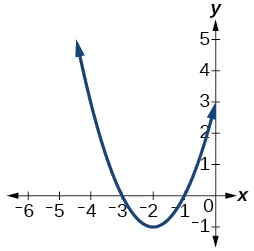For the following exercises, determine whether each function below is even, odd, or neither.

57.

$f(x)=3 x 4 f(x)=3 x 4$

58.

$g(x)= x g(x)= x$

59.

$h(x)= 1 x +3x h(x)= 1 x +3x$

For the following exercises, analyze the graph and determine whether the graphed function is even, odd, or neither.

60.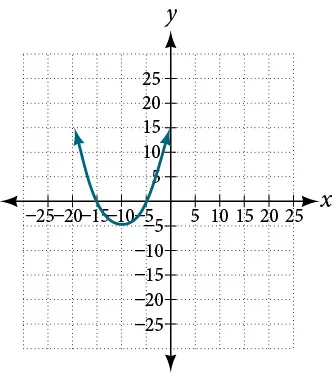61.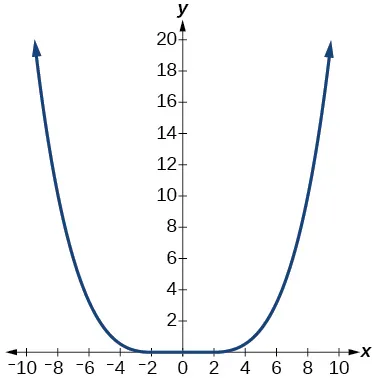62.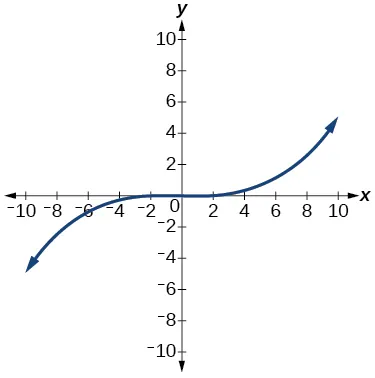##### Absolute Value Functions

For the following exercises, write an equation for the transformation of $f(x)=| x |. f(x)=| x |.$

63.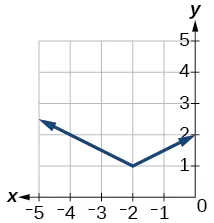64.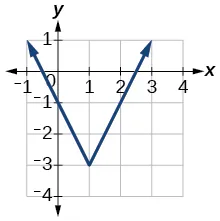65.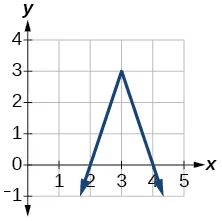For the following exercises, graph the absolute value function.

66.

$f(x)=| x−5 | f(x)=| x−5 |$

67.

$f(x)=−| x−3 | f(x)=−| x−3 |$

68.

$f(x)=| 2x−4 | f(x)=| 2x−4 |$

##### Inverse Functions

For the following exercises, find $f −1 (x) f −1 (x)$ for each function.

69.

$f(x)=9+10x f(x)=9+10x$

70.

$f(x)= x x+2 f(x)= x x+2$

For the following exercise, find a domain on which the function $f f$ is one-to-one and non-decreasing. Write the domain in interval notation. Then find the inverse of $f f$ restricted to that domain.

71.

$f(x)= x 2 +1 f(x)= x 2 +1$

72.

Given $f( x )= x 3 −5 f( x )= x 3 −5$ and $g(x)= x+5 3 : g(x)= x+5 3 :$

1. Find and $g(f(x)). g(f(x)).$
2. What does the answer tell us about the relationship between $f(x) f(x)$ and $g(x)? g(x)?$

For the following exercises, use a graphing utility to determine whether each function is one-to-one.

73.

$f(x)= 1 x f(x)= 1 x$

74.

$f(x)=−3 x 2 +x f(x)=−3 x 2 +x$

75.

If $f( 5 )=2, f( 5 )=2,$ find $f −1 (2). f −1 (2).$

76.

If $f( 1 )=4, f( 1 )=4,$ find $f −1 (4). f −1 (4).$

Order a print copy

As an Amazon Associate we earn from qualifying purchases.